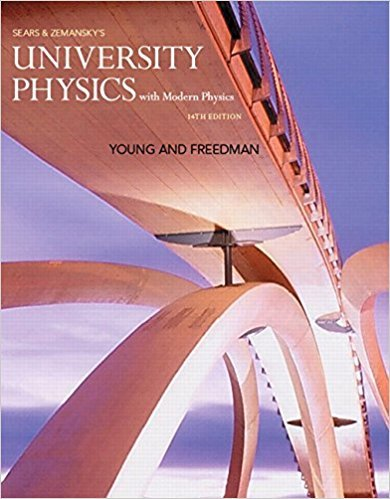×
×

# Solution: Figure E2.12 shows the velocity of a solar-poweredISBN: 9780321973610 228

## Solution for problem 2.12 Chapter 2

University Physics with Modern Physics (1) | 14th Edition

• Textbook Solutions
• 2901 Step-by-step solutions solved by professors and subject experts
• Get 24/7 help from StudySoup virtual teaching assistantsUniversity Physics with Modern Physics (1) | 14th Edition

4 5 1 301 Reviews
31
1
Problem 2.12

Figure E2.12 shows the velocity of a solar-powered car as a function of time. The driver accelerates from a stop sign, cruises for 20 s at a constant speed of 60 km/h, and then brakes to come to a stop 40 s after leaving the stop sign. (a) Compute the average acceleration during these time intervals: (i) t = 0 to t = 10 s; (ii) t = 30 s to t = 40 s; (iii) t = 10 s to t = 30 s; (iv) t = 0 to t = 40 s. (b) What is the instantaneous acceleration at t = 20 s and at t = 35 s?

Step-by-Step Solution:
Step 1 of 3

Introduction In this lab we studied two­dimensional motion. The purpose is to find horizontal and vertical acceleration and velocity of a ball moving in a plane. We fired a ball out of a Projectile Launcher on to a Pasco timer plate and calculated data. Procedure First, we predicted the horizontal and vertical accelerations of the shadows after the ball leaves the thrower’s hand. To test...

Step 2 of 3

Step 3 of 3

##### ISBN: 9780321973610

The full step-by-step solution to problem: 2.12 from chapter: 2 was answered by , our top Physics solution expert on 01/09/18, 07:46PM. This textbook survival guide was created for the textbook: University Physics with Modern Physics (1), edition: 14. Since the solution to 2.12 from 2 chapter was answered, more than 241 students have viewed the full step-by-step answer. The answer to “Figure E2.12 shows the velocity of a solar-powered car as a function of time. The driver accelerates from a stop sign, cruises for 20 s at a constant speed of 60 km/h, and then brakes to come to a stop 40 s after leaving the stop sign. (a) Compute the average acceleration during these time intervals: (i) t = 0 to t = 10 s; (ii) t = 30 s to t = 40 s; (iii) t = 10 s to t = 30 s; (iv) t = 0 to t = 40 s. (b) What is the instantaneous acceleration at t = 20 s and at t = 35 s?” is broken down into a number of easy to follow steps, and 111 words. University Physics with Modern Physics (1) was written by and is associated to the ISBN: 9780321973610. This full solution covers the following key subjects: . This expansive textbook survival guide covers 44 chapters, and 4574 solutions.

Unlock Textbook Solution